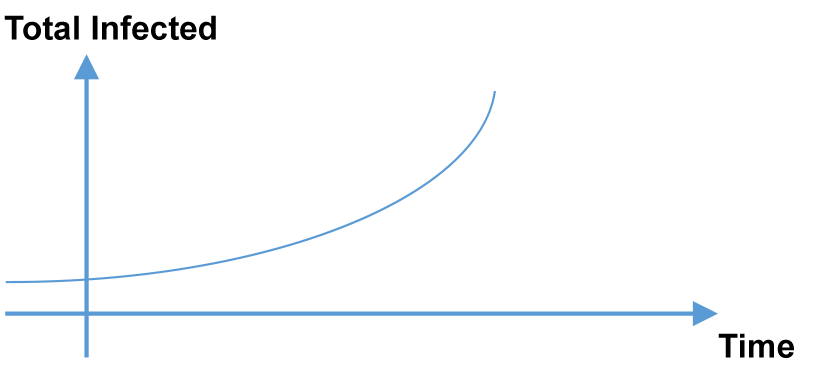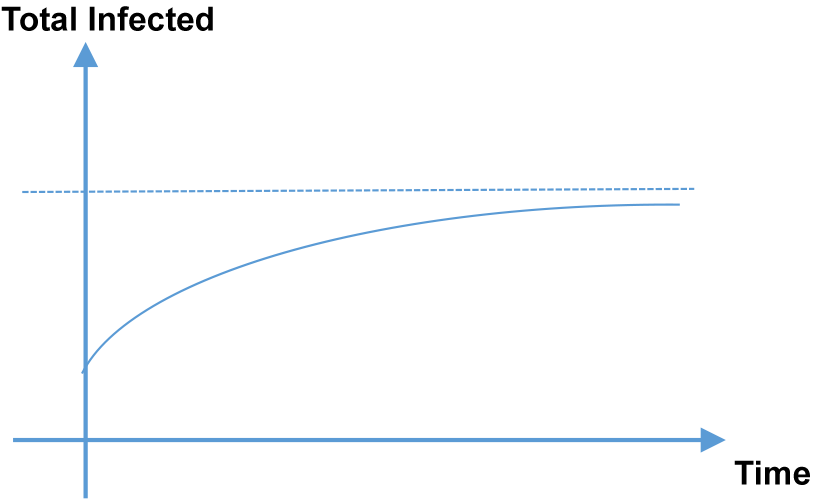Home > Blog > Applicability of H2 mathematics to the COVID-19 Situation

# Applicability of H2 mathematics to the COVID-19 Situation

May 22, 2020 | By Yi KaiNot only do H2 math and H2 math tuition teach you how to solve questions during the examinations, they also help you gain certain mathematical life skills. You could learn how to better manage your finances by choosing a bank that offers a greater return on interest through the use of differential equations or you could also learn how to maximise the use of your time using optimisation from calculus. Recently, arithmetic progression and geometric progression from A Level math tuition could actually help you understand the Covid-19 situation around you and why a lockdown is necessary.

Let’s say there is one infected person in the country on the first day and if the infected person was to infect another 3 people on the second day. Subsequently, on the second day the 3 infected people from the previous day would continue to each infect 3 other people. This would cause the total infected to be:

Day 1 total infected =1
Day 2 total infected =1+3
Day 3 total infected =1+3+9
Day 4 total infected =1+3+9+27
Till on the nth day,
Total infected= 1+3+32+33 +…+3(n-1)
This seems all too familiar to those who are studying h2 math and those who attend a level math tuition. It would form a sum of geometric progression terms. So the total on the nth day would be:

In=
3n-1
/
3-1
=
1
/
2
(3n-1)As you could see the, total infected would increase exponentially to infinity and the whole population would be infected. However, if on average a person was to infect other people, where 0<µ<1, then the story would be different.

Day 1 total infected =1
Day 2 total infected =1+µ
Day 3 total infected =1+µ+µ2
Day 4 total infected =1+µ+µ23
And on the nth day,

In=
1-µn
/
1-µAs you could see the number of infected would increase but as the time goes by the rate of increase would slow down. H2 Math students and any other math A level math student would tell you that the total infected would tend to 1/(1-µ) as the value n tends to infinity. This is because the value of µn would tend to zero as the value of n tend to infinity.

Some students who have attended H2 math lessons or A Level math tuition might point out that there are also people who recover from the infection and no longer would be able to infect others. Assuming the number of people who have recovered from the virus is k patients per day and this starts on the second day of infections. Say that each infected person would infect another α people per day. Then on each day, the number of infected would be (α+1) times the number of infected on the previous day. Let β=1+α.

Day 1, I1=1
Day 2 I2=β (1)- k
Day 3 = β (β-k)- k= β2 k(1+β)
Day 4 = β [β2k(1-β)]- k = β3 k (1+β+β2)

Day n=βnk(1+β+β<sup2+…+ βn-1]

n
k (1-β^n )
/
(1-β)

From the above, to reduce the number of infected as far as possible on the nth day, we would have to keep the value of β small and hence that goes to say that we would have to lower the infection rate, α . This justifies why the government might want to impose a lockdown. Moreover, we would also want to increase the rate of recovery, k, and with α being small, 1-βn would be larger and 1/(1-β) would also be larger, amplifying the total reduction for the nth day.

However, reality might not match such a model. Definitely, the recovery rate is not a constant in Singapore and varies with the amount of spare capacity within our healthcare systems. We could then model it as inversely proportional to the number of infected, assuming that all infected do seek medical treatment.

Hence, as you can see, H2 math lessons do give valuable insights to model the world around us instead of just teaching you how to ace the examinations. Thus, we should face the subject with a positive attitude and see it as a tool to analyse the world around us. Who knows, maybe it would improve your life.